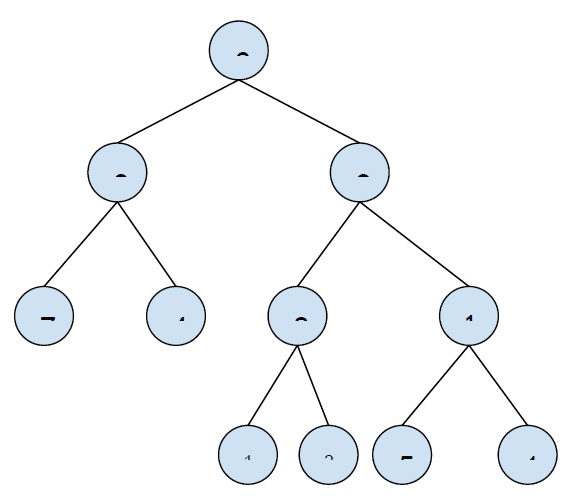# Print all nodes between two given levels in Binary Tree in C++

In this problem, we are given a binary tree and two levels in the tree (upper and lower) and we have to print all nodes between upper and lower levels of the tree.

The binary tree is a special tree whose each node has at max two nodes (one/two/none).

Let’s take an example to understand the problem −upper − 1

lower − 3

Output

6
3 9
7 4 8 10

To solve this problem, we have to print nodes of the tree at a given level. We will call a recursive function using a loop from the upper to lower level in the tree.

This algorithm is simple but is more complex of order n2.

A more effective solution is doing inorder traversal and using a queue. And print nodes within the given upper and lower levels.

Program to implement our solution −

## Example

Live Demo

#include <iostream>
#include <queue>
using namespace std;
struct Node{
int key;
struct Node* left, *right;
};
void printNodesAtLevel(Node* root, int low, int high){
queue <Node *> Q;
Node *marker = new Node;
int level = 1;
Q.push(root);
Q.push(marker);
while (Q.empty() == false){
Node *n = Q.front();
Q.pop();
if (n == marker){
cout << endl;
level++;
if (Q.empty() == true || level > high) break;
Q.push(marker);
continue;
}
if (level >= low)
cout<<n->key<<" ";
if (n->left != NULL) Q.push(n->left);
if (n->right != NULL) Q.push(n->right);
}
}
Node* insertNode(int key){
Node* temp = new Node;
temp->key = key;
temp->left = temp->right = NULL;
return (temp);
}
int main() {
struct Node *root = insertNode(6);
root->left = insertNode(3);
root->right = insertNode(9);
root->left->left = insertNode(7);
root->left->right = insertNode(4);
root->left->right->left = insertNode(8);
root->left->right->right = insertNode(10);
root->left->right->right->left = insertNode(5);
root->left->right->right->right = insertNode(1);
root->left->right->left->left = insertNode(14);
root->left->right->left->right = insertNode(26);
int upper = 3;
int lower = 1;
cout << "Level wise Nodes between level "<<lower<<" and "<<upper<<" are \n";
printNodesAtLevel(root, lower, upper);
return 0;
}

## Output

Level wise Nodes between level 1 and 3 are
6
3 9
7 4